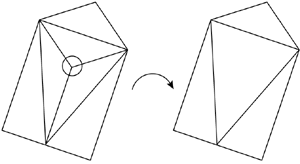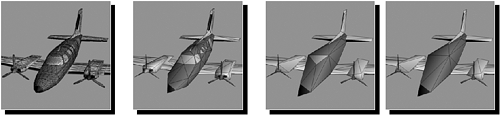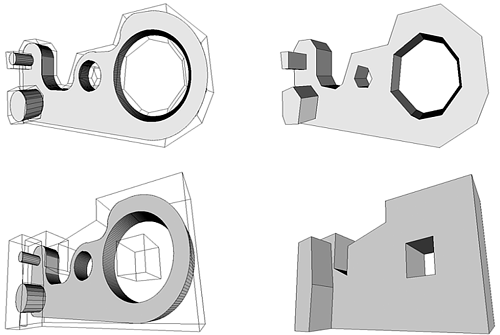# Triangle Reduction

Another family of geometrical algorithms we will review is triangle reduction methods, which are used to create variable-resolution meshes from a base mesh. Although some games never use triangle reduction or use it at the modeling stage, many others find it useful to perform some kind of software triangle reduction. Some popular reasons for this are

• Selecting a level of detail for an object that can be up close or far away

• Using lower resolution meshes to compute shadows

• Tuning the game to the player's hardware capabilities at load time

Whichever your case, triangle reduction policies can be implemented either as a preprocess at load time, assuming computational cost is relatively unimportant, or as a real-time process, computing the reduced mesh in real time. Obviously, the algorithms will be different depending on your choice. Triangle reduction is not a simple process, and thus trying to compute a good multiresolution mesh in real time will definitely need some careful planning.

#### Vertex Collapsing

Vertex collapsing involves eliminating vertices from the base mesh using the criteria of coplanarity with neighboring vertices. It requires reconstructing the mesh once the vertex has been deleted.

Fundamentally, we will need to arrange geometrical data in memory so we can traverse the structure using both geometric and topological relationships. We will need to quickly determine which edges are connected to a certain vertex or compute by-face normals for a given face. The core of the algorithm for each vertex is to compare the per-face normals for those faces our original vertex belongs to. If the difference in the normals is below a certain threshold, we will delete the vertex from the data structure, and by traversing those edges that have actually survived, reconstruct the base mesh (see Figure 22.9). By iterative application, we will reach lower triangle rates, and thus higher performance.

##### Figure 22.9. Vertex collapse. Left, original mesh. Right, mesh after the central vertex has been deleted.#### Edge Collapsing

An interesting but similar approach works not on vertices, but on edges. An edge is shared by at most two triangles. Then, it is all a matter of detecting those edges whose neighboring triangles have a large index of coplanarity. If a certain threshold is met, we can delete the edge and weld the vertices at both sides of the edge together. To do so we remove the vertices at both ends of the edge and create a new one located in the midpoint between the two and on the axis formed by them. An example of edge collapse is shown in Figure 22.10.

##### Figure 22.10. Edge collapse. Left, original mesh. Right, mesh after the highlighted edge has been deleted.#### Progressive Meshes

One of the most popular real-time triangle reduction algorithms is progressive meshes, introduced by Hugues Hoppe in 1996. The algorithms we have seen so far are designed to build a low-resolution mesh from a base, high-quality model. Progressive meshes go one step beyond and allow real-time continuous level-of-detail (LOD), so we can increase and decrease triangle counts as needed and always have a model whose complexity is just right for each moment. All these benefits come from preprocessing the mesh so both the initial topology and constructive description are stored. Then, the run-time algorithm is simple, so it can be used for games.

Progressive meshes start with a base mesh, which is reduced, one edge at a time, using a variation of the edge collapse strategy outlined earlier. As we have already seen, given a mesh Mn, we can compute mesh Mn-1 by performing a one edge collapse. This removes one vertex and two faces exactly. Now, notice that we can revert the process and reconstruct Mn from Mn-1 if we store the information of the collapse: the positions of the two vertices and the connectivity of each one. This means the collapse operation is invertible. We will call the inverse operation a vertex split, because it takes one (collapsed) vertex and divides it in two.

Then, by sequencing vertex splits, we can represent any mesh by means of

• A low-quality mesh as an initial M0

• The ordered sequence of vertex splits we need to perform to move from M0 to the high-quality Mn

This is the core of the progressive mesh concept. We store a low-quality mesh and the constructive method to generate higher quality versions. Then, at runtime, we use these two structures to build a mesh that is just perfect for our needs. Meshes usually move closer or farther progressively, so we will just need to perform vertex splits or merges every few frames because sudden changes in resolution are not frequent. A series of progressive meshes is depicted in Figure 22.11, showcasing the progression from a high-quality mesh to a very low-resolution mesh.

##### Figure 22.11. Progressive meshes. The leftmost mesh is progressively reduced until the rightmost version is computed.To build a progressive mesh, we must start with the high-quality version.

Progressive meshes suffer from much less popping than other methods. In the end, triangles are continually added, so the changes in appearance are very small. But sometimes we notice vertices moving, which can become a bit annoying. An improvement over the initial progressive mesh idea can be implemented to completely avoid those annoying pops and ensure an always-perfect appearance. The enhancement, called geomorphing, works by considering a vertex split as a continuous process, as opposed to a discrete one. When we need to split a vertex in two, we will do this progressively by beginning with two vertices that are located where the original one was, and then moving them along the axis until they reach the final position, providing a higher smoothness to our LODs.

Another, quite interesting advantage of progressive meshes comes from their suitability to the online medium. This representation makes meshes ideal for massively multi player games where content must be downloaded from a host in real time during the course of the game. Intuitively, imagine that a player is traversing the game world and happens to reach a zone he's never been to before, with new geometry pieces, like a castle, for example. Logically, the castle will appear from the horizon as we approach it. It is only a few pixels across and immersed in fog. Thus, we can begin by downloading M0, which is small and therefore efficient for transmission over a communications line. Quality will not be very good, but that's not necessary at this stage anyway. Then, we can start sending the sequence of vertex splits, so the player can always get the best representation as he moves closer to the new geometry piece. Transmitting vertex splits is very efficient because the amount of data involved is relatively small. Once we end the transmission of the whole sequence, we will have the final version of the castle persistently in memory and on our hard drive, because progressive meshes are lossless. This is one of the reasons progressive meshes work with low-quality meshes and vertex splits instead of working with high-quality versions and vertex merges. Many online games build their graphics pipelines around a progressive mesh processor for locations and items, and a terrain renderer that supports large data sets.

#### Nonconservative Triangle Reduction

All the algorithms we have seen so far belong to a global family called conservative triangle reduction algorithms. If you analyze them globally, they all start with the base mesh and try to detect unimportant elements, whether it's vertices, faces, edges, and so on. But does that really define a low-detail object? Not really. What defines a low-detail representation of an object is that, seen from the right distance, it looks very similar to the original version. Reducing triangles by selecting unimportant ones is just one of the ways to face the problem. We could simply choose to start from scratch and build a whole new mesh that represents the original one, but has fewer triangles. This new method does not guarantee we will preserve part of the original geometry data. In fact, we will generally throw it away and replace it with a totally new set of vertices, edges, and so on. This new representation will, however, look very similar to the original one when seen from a distance.

Nonconservative algorithms generally provide much better results, especially for dramatic triangle reductions where conservative algorithms fail to find unimportant data. If you need to reduce a mesh by 50 percent or more, it is likely that you will reach a point where all vertices will contribute to the object's shape, and trying to reduce even more will simply collapse some areas in the mesh and spoil the overall look. As an example, compare the four images in Figure 22.12. The top-left image is the original mesh, consisting of 1,366 triangles. The bottom-right image is the result of a nonconservative algorithm, reducing the mesh to only 21 triangles (that's 1.5% of the original cost). Notice how the algorithm has completely replaced the original object with a new one, whose shape more or less resembles it. A conservative algorithm used to that degree of reduction would simply have destroyed the object's appearance completely.

##### Figure 22.12. Nonconservative triangle reduction.As an example of nonconservative triangle reduction, take the Trihedral Discretized Polyhedra Simplification (TDPS) algorithm presented by Andujar in his doctoral dissertation (reference and links in Appendix E, "Further Reading"). This algorithm reduces triangle counts by first analyzing the space occupancy and then rebuilding a new object based on this space occupancy information. Thus, it offers much better results, especially when trying to create dramatic triangle reductions. The core idea is quite simple: Construct an octree based on the space occupancy of the mesh. Octree nodes are marked as fully outside, fully inside, and on the edge. These last nodes are the ones we will recurse until a desired geometry detail level is reached. The octree stores data in the leaves as well as in the internal nodes. Looking at the leaves, we will discover the detailed space occupation information, and as we move closer to the root, shapes become coarser. Then, at runtime, a threshold is fixed so we can limit the depth of the octree traversal. We scan the octree, and as we reach the limit of our traversal with regard to the maximum threshold, we apply a marching cubes approach so we tessellate each cell into the minimum number of triangles, which is usually two. This way, a different threshold generates a different LOD, but all of them are based on space occupancy, not actual geometry. Check out the pictures in Figure 22.12 and notice how the shape-based triangle reduction policy delivers very good results where traditional decimation methods would fail.Core Techniques and Algorithms in Game Programming2003
ISBN: N/A
EAN: N/A
Year: 2004
Pages: 261